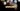# Leetcode | Solution of Jewels and Stones

March 24th, 2020
|

In this post, we will solve problem jewels and stones from leetcode and compute the time and space complexities. Let's begin.

# Problem Statement

The question can be found at leetcode jewels and stones problem.

The problem states that we need to determine the number of jewels in a list of stones based on given constraints.

# Constraints and challenges

• The list of all the jewels are unique
• The characters are case sensitive i.e. "a" is not equal to "A"

# Solution

We will discuss a solution using a JavaScript Set and a simple for loop.

The approach is as follows

• we will add all the characters from the list of jewels in the set
• we will declare a counter to keep track of the number of jewels in stones
• next, we'll loop over all the stones and check for their presence in jewel set
• if the stone is a jewel, we'll increase the counter
• once we've looked at all the stones, we'll jump out of the loop and return the counter.

We have discussed the approach, I urge you to go ahead on leetcode and give it another try.If you are here, it means something went wrong in implementation or you are just too lazy. In any case, let's see a simple implementation of the above logic.
``````var numJewelsInStones = function (J, S) {
const set = new Set(J.split(""));
let count = 0;

for (let i = 0; i < S.length; i++) {
if (set.has(S[i])) {
count++;
}
}

return count;
};``````

If you notice, there are multiple moving parts on line 2

• We are converting the string `J` into an array
• we are passing the array into the `Set constructor`
• we can do this because the set constructor can take any iterable

If we had duplicates, we would have to store the jewels in a map as opposed to a set, since set doesn't have any duplicates. Also, if the jewels were case insensitive, we would have to convert both the strings in either lowercase or upper case to maintain consistency.

``````Status: Accepted
Runtime: 56ms
Memory: 34MB``````

## Time and space complexity

### Time complexity

We are looping over the string `S` (stones) once, so time complexity would be O(len S) or O(n).

### Space complexity

We are using extra space to keep all the `jewels in a Set`, thus, space complexity would be O(len J) or O(n).

# Summary

So, we solved the jewels and stones problem by using a simple while loop and a set. Finally, calculated the time and space complexities.

I hope you enjoyed solving this question. This is it for this one, complete source code for this post can be found on my Github Repo. Will see you in the next one.